## Operator CastKernel

Library: Base

The operator CastKernel enables the re-organisation of incoming data with respect to parallelism and kernel size. For re-interpretation, change the kernel size at the output link. For example, an input link is configured with two kernel rows and two kernel columns and a parallelism of four. The CastKernel operator gives you the possibility to interpret these as four kernel rows and four kernel columns at parallelism 1 or as one kernel row and two kernel columns at parallelism 8, etc. The constraint of the operator CastKernel is that the product of kernel row and kernel column and parallelism must be identical for the input and the output link.

The following examples illustrate the conversion of kernel configuration and parallelism performed by the operator. The pseudo-code below the illustrations also describes the conversion pattern.

Example 1: Conversion from kernel size (col x row) 3x1 to kernel size 1x3 while keeping parallelism 2: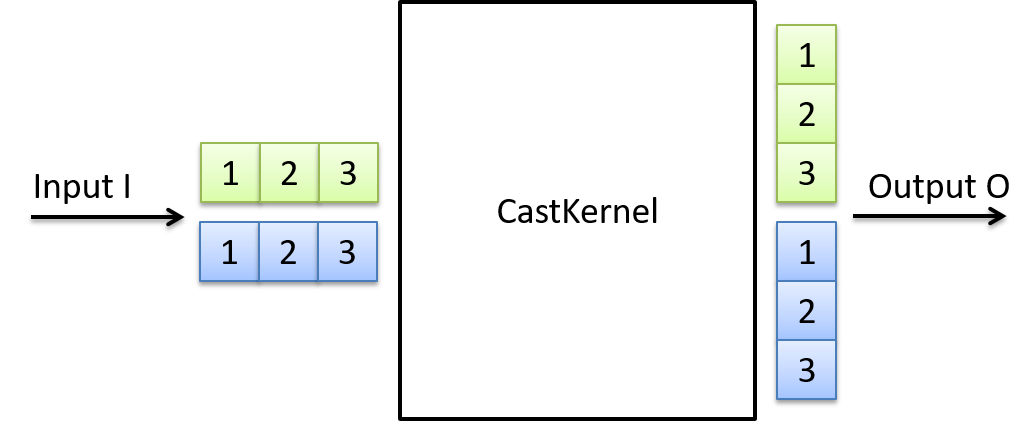Example 2: Conversion from parallelism 2 and kernel size (col x row) 2x3 to parallelism 3 and kernel size 2x2: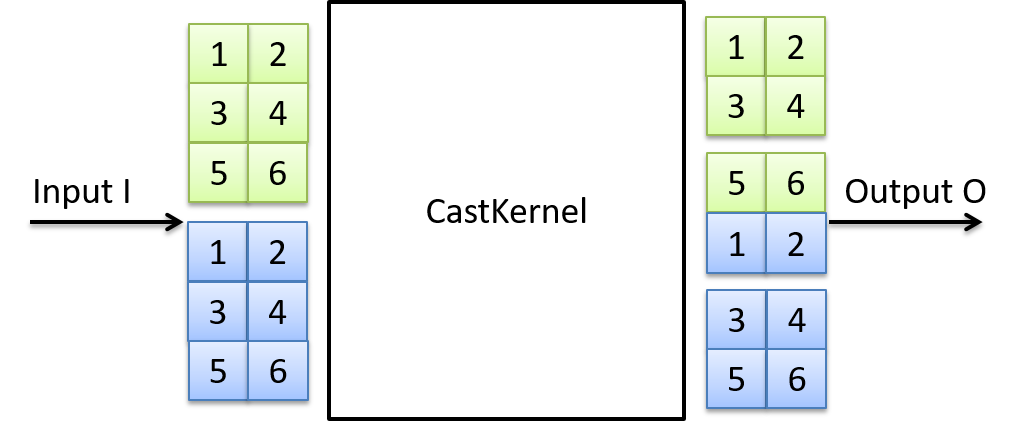Example 3: Conversion from parallelism 1 and kernel size (col x row) 3x2 to parallelism 6 and kernel size 1x1: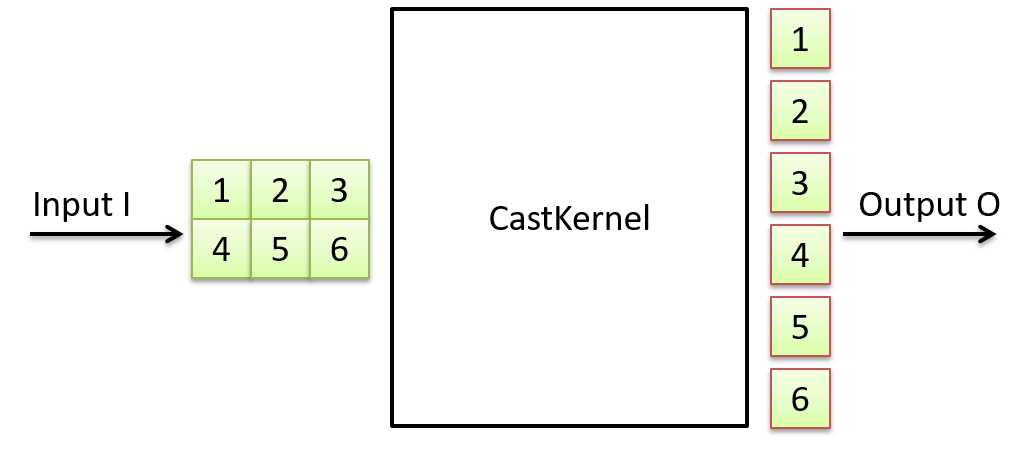Note that the operator will change the width of the images.

The mapping follows the following pseudo-code:

```
pi = 0
ri = 0
ci = 0
for p in 0 to P-1
for r in 0 to R-1
for c in 0 to C-1
O[p][r][c] = I[pi][ri][ci]
ci = ci + 1
if ci >= Ci then ci = 0, ri = ri + 1
if ri >= Ri then ri = 0, pi = pi + 1

```

The pseudo-code has the following meaning:

Pseudo-code Meaning
p Output-Parallel-Index
pi Input-Parallel-Index
r Output-Kernel-Row-Index
ri Input-Kernel-Row-Index
c Output-Kernel-Column-Index
co Output-Kernel-Column-Index
Pi Input-Parallelism
P Output-Parallelism
Ri Input-Kernel-Rows
R Input-Kernel-Columns
Ci Input-KernelColumns
C OutputKernelColumns

Table 21. Explanation of pseudo-code

The operation performed can be expressed by a set of existing operators, such as SplitKernel, MergeKernel, SplitParallel and MergeParallel: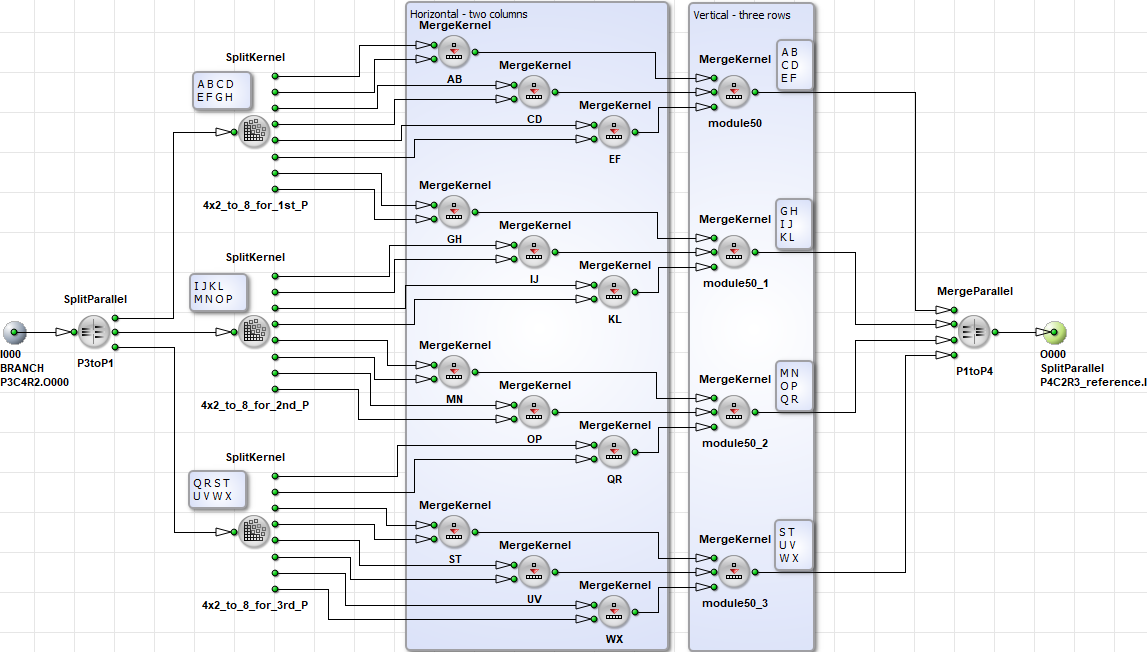In this example, the input configuration of four kernel columns and two kernel rows at parallelism 3 is re-organised to two kernel columns and three kernel rows at parallelism 4. The same can be achieved via the operator CastKernel by configuring the output link respectively: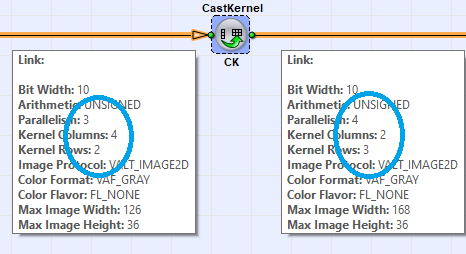### I/O Properties

Property Value
Operator Type O
Input Link I, data input
Output Link O, data output

### Supported Link Format

Bit Width [1, 64]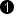As I
Arithmetic {Unsigned, signed} As I
Parallelism Any Auto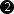Kernel Columns Any Any
Kernel Rows Any Any
Img Protocol {VALT_IMAGE2D, VALT_LINE1D, VALT_PIXEL0D} As I
Color Format Any As I
Color Flavor Any As I
Max. Img Width Any Auto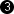Max. Img Height Any As I
 The range for bit width is: For unsigned inputs: [1, 64] For signed inputs: [2, 64] For unsigned color inputs: [3, 63] For signed color inputs: [6, 63] The output parallelism is determined by the input parallelism , the input kernel size and the output kernel size : where denotes the kernel rows and denotes the kernel columns. The output maximum image width is determined by the input maximum image width, the output parallelism and the input parallelism by:

None